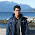## Wednesday, August 17, 2011

### Maximum Triangle Area (Part 1)

Today I'll discuss about another interesting problem. Though the analysis is big, hopefully you'll find it interesting.

Problem name: Maximum Triangle Area
Problem description:
Given n distinct points on a plane, your task is to ﬁnd the triangle that have the maximum area, whose vertices are from the given points.

The most obvious solution is trying all possible triangle and find out the maximum area. The complexity of that algorithm is O(n^3). Which is huge as n can be as large as 50000.

So what can we do now? An interesting fact is, we can solve this problem by only considering the points of the convex hull for the given set of points. This will work because, if there is a triangle which has a vertex A which is not in the boundary of the convex hull, there will be always a point X in convex hull such that, by moving A to X we can increase the area of the triangle. Let's see an example -We have a set of points {A, B, C, D, E, F, G, H, I}. The convex hull of these points consists of {D, E, F, G, H, I}. But let's claim that the largest triangle using these points is ABC. None of it's vertex is in convex hull. Let's say, BC is the base of this triangle and AP (perpendicular distance from A to BC) is the height of the triangle. So, the area of the triangle is 0.5 * BC * AP. Now draw a line parallel to the BC which goes through A. As A is inside the convex hull, you will find at least one vertex from the convex hull which is on the other side of the parallel line. Here in our example, we found D and BC are in the opposite side of the parallel line, so perpendicular distance from D to BC (DQ) is greater than AP. Hence, 0.5 * BC * AB < 0.5 * BC * DQ. So, triangle area DBC > triangle area ABC. See the following picture to make it clear -Now, we got a triangle DBC which has larger area than ABC, but yet we have two vertices in it which are not in the convex hull. Now in the similar way considering DB as a base, and drawing a parallel line of DB which goes through C, we will find that area of DBF is greater than area of DBC. In the next step we can easily show again, that area of DBH is greater than the area of DBF. So we found that, maximum area triangle can be found using only convex hull vertices.But if all of the given points is in the convex hull, our algorithm is still O(n ^ 3). Using the convex hull property we can improve it. Now our problem is finding the largest triangle using the vertices of a convex polygon. Let's say we have n vertices 0 to n - 1. We will try to find out three vertices A, B, C in clockwise direction from these vertices which will form the maximum triangle.

Now for a given AB, rather than iterating all of the points between B and A, to find the C, we can use ternary search to find C, as if we consider AB as a base, and iterate from the next point of B one by one, the height (of the triangle ABC) will increase in every step up to a certain vertex, and after that height will decrease in every step. This way, we can ternary search the point C for every possible AB, and the complexity will become (n ^ 2 log n), which is still not enough.

As, the analysis becoming large and large, we'll discuss the other improvements in the next part.

(Special thanks to Anna Fariha for helping me understanding some proofs of this analysis.)

1.Great post! Eagerly waiting for the next part!

2.Great stuff, loved to read. I wonder if you can post something for beginners like me. A post that gives an idea of elementary things about algorithms, references and direction.

I hope you'll continue writing in this blog and share your knowledge.

3.I tried this problem but left without success. Very lucid analysis but I could reach at this point. Now I am eagerly waiting for the next part.

4.Thanks All. Next post is up.
@maruf.hasan: I have a plan about that also to write something for beginner in (may be near) future.

5.Hey! This problem came in the National Programming Camp 2013 selection test. Too bad I was only able to provide the n^3 solution.